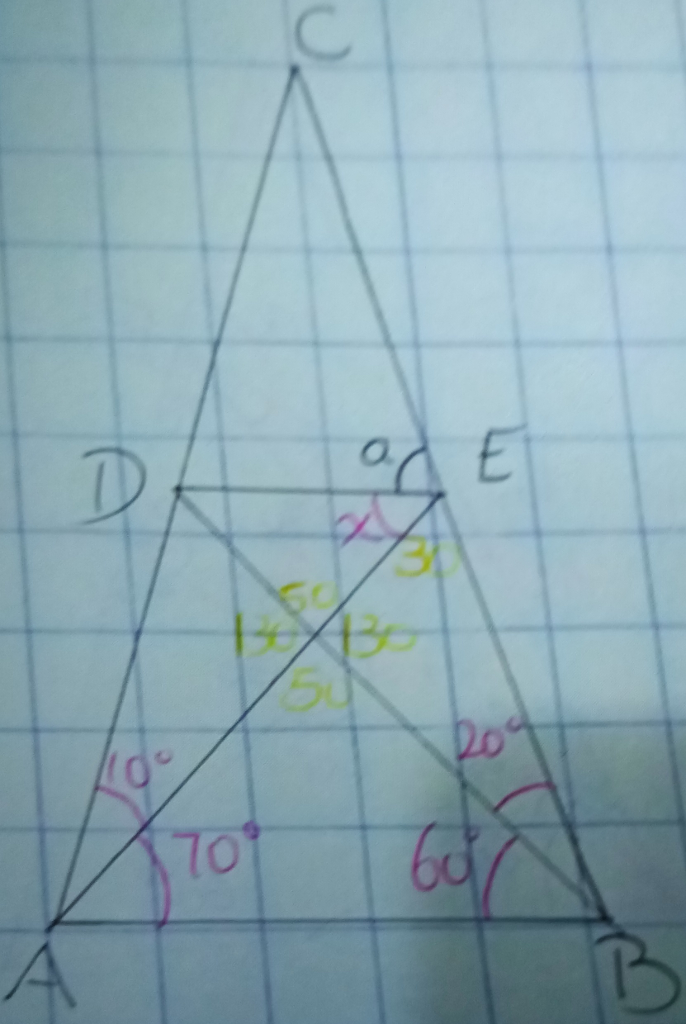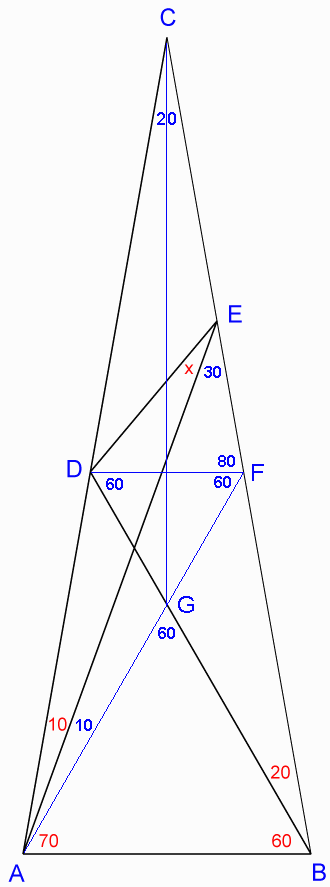##本區搜索:
Yahoo!字典# [Core] 幾何難題

[隱藏]

## 幾何難題TOP

## 回覆 1# cindylee2013 的帖子

cannot see the picture clearly

TOP

## 回覆 1# cindylee2013 的帖子1. Calculate some known angles:
ACB = 180-(10+70)-(60+20) = 20°
AEB = 180-70-(60+20) = 30°

2. Draw a line from point D parallel to AB, labeling the intersection with BC as a new point F and conclude:
DCF  ACB
CFD = CBA = 60+20 = 80°
DFB = 180-80 = 100°
CDF = CAB = 70+10 = 80°
BDF = 180-100-20 = 60°

3. Draw a line FA labeling the intersection with DB as a new point G and conclude:
AFD = BDF = 60°
DGF = 180-60-60 = 60° = AGB
GAB = 180-60-60 = 60°
DFG (with all angles 60°) is equilateral
AGB (with all angles 60°) is equilateral
4. CFA with two 20° angles is isosceles, so FC = FA

5. Draw a line CG, which bisects ACB and conclude:
ACG  CAE
FC-CE = FA-AG = FE = FG
FG = FD, so FE = FD

6. With two equal sides, DFE is isosceles and conclude:
DEF = 30+x = (180-80)/2 = 50